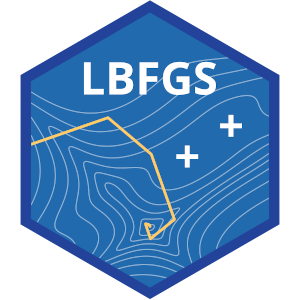LBFGS++
Searching...
No Matches
LBFGS++UPDATE on 2020-03-06: LBFGS++ now includes a new L-BFGS-B solver for box-constrained optimization problems. Check the example below for its usage.

LBFGS++ is a header-only C++ library that implements the Limited-memory BFGS algorithm (L-BFGS) for unconstrained minimization problems, and a modified version of the L-BFGS-B algorithm for box-constrained ones.

The code for the L-BFGS solver is derived and modified from the libLBFGS library developed by Naoaki Okazaki.

LBFGS++ is implemented as a header-only C++ library, whose only dependency, Eigen, is also header-only.

# A Quick Example

To use LBFGS++, one needs to first define a functor to represent the multivariate function to be minimized. It should return the objective function value on a vector x and overwrite the vector grad with the gradient evaluated on x. For example we could define the Rosenbrock function in the following way:

#include <Eigen/Core>
#include <iostream>
#include <LBFGS.h>
using Eigen::VectorXd;
using namespace LBFGSpp;
class Rosenbrock
{
private:
int n;
public:
Rosenbrock(int n_) : n(n_) {}
double operator()(const VectorXd& x, VectorXd& grad)
{
double fx = 0.0;
for(int i = 0; i < n; i += 2)
{
double t1 = 1.0 - x[i];
double t2 = 10 * (x[i + 1] - x[i] * x[i]);
grad[i + 1] = 20 * t2;
fx += t1 * t1 + t2 * t2;
}
return fx;
}
};

Then we just need to set up parameters, create solver object, provide initial guess, and then run the minimization function.

int main()
{
const int n = 10;
// Set up parameters
param.epsilon = 1e-6;
param.max_iterations = 100;
// Create solver and function object
LBFGSSolver<double> solver(param);
Rosenbrock fun(n);
// Initial guess
VectorXd x = VectorXd::Zero(n);
// x will be overwritten to be the best point found
double fx;
int niter = solver.minimize(fun, x, fx);
std::cout << niter << " iterations" << std::endl;
std::cout << "x = \n" << x.transpose() << std::endl;
std::cout << "f(x) = " << fx << std::endl;
return 0;
}
Scalar epsilon
Definition: Param.h:88

The example can then be compiled and run.

$g++ -I/path/to/eigen -I/path/to/lbfgspp/include -O2 example.cpp$ ./a.out
23 iterations
x =
1 1 1 1 1 1 1 1 1 1
f(x) = 1.87948e-19

You can also use a different line search algorithm by providing a second template parameter to LBFGSSolver. For example, the code below illustrates the bracketing line search algorithm (contributed by @DirkToewe).

int main()
{
const int n = 10;
// Set up parameters
param.epsilon = 1e-6;
param.max_iterations = 100;
// Create solver and function object
Rosenbrock fun(n);
// Initial guess
VectorXd x = VectorXd::Zero(n);
// x will be overwritten to be the best point found
double fx;
int niter = solver.minimize(fun, x, fx);
std::cout << niter << " iterations" << std::endl;
std::cout << "x = \n" << x.transpose() << std::endl;
std::cout << "f(x) = " << fx << std::endl;
return 0;
}

# Box-constrained Problem

If the parameters to be optimized have simple bounds, then the L-BFGS-**B** solver class LBFGSBSolver can be used. The code is very similar to that of LBFGSSolver. Below is the same Rosenbrock example, but we require that all variables should be between 2 and 4.

#include <Eigen/Core>
#include <iostream>
#include <LBFGSB.h> // Note the different header file
using Eigen::VectorXd;
using namespace LBFGSpp;
class Rosenbrock
{
private:
int n;
public:
Rosenbrock(int n_) : n(n_) {}
double operator()(const VectorXd& x, VectorXd& grad)
{
double fx = 0.0;
for(int i = 0; i < n; i += 2)
{
double t1 = 1.0 - x[i];
double t2 = 10 * (x[i + 1] - x[i] * x[i]);
grad[i + 1] = 20 * t2;
fx += t1 * t1 + t2 * t2;
}
return fx;
}
};
int main()
{
const int n = 10;
// Set up parameters
LBFGSBParam<double> param; // New parameter class
param.epsilon = 1e-6;
param.max_iterations = 100;
// Create solver and function object
LBFGSBSolver<double> solver(param); // New solver class
Rosenbrock fun(n);
// Bounds
VectorXd lb = VectorXd::Constant(n, 2.0);
VectorXd ub = VectorXd::Constant(n, 4.0);
// Initial guess
VectorXd x = VectorXd::Constant(n, 3.0);
// x will be overwritten to be the best point found
double fx;
int niter = solver.minimize(fun, x, fx, lb, ub);
std::cout << niter << " iterations" << std::endl;
std::cout << "x = \n" << x.transpose() << std::endl;
std::cout << "f(x) = " << fx << std::endl;
return 0;
}

Note that we also allow infinite values for the lower and upper bounds. In such cases one can define ub[i] = std::numeric_limits<double>::infinity(), for example.

# Documentation

The API reference page contains the documentation of LBFGS++ generated by Doxygen.